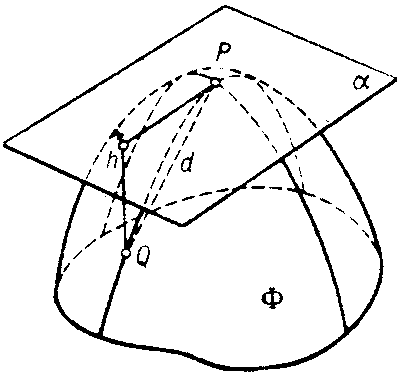# Osculating paraboloid

(diff) ← Older revision | Latest revision (diff) | Newer revision → (diff)
Jump to: navigation, search

of a surface at a point $P$

The paraboloid that reproduces the shape of the surface near this point up to variables of the second order of smallness with respect to the distance from $P$. Let $\Phi$ be a paraboloid (see Fig.) with vertex $P$ and tangent to the surface at this point, and let $h$ and $d$ be the distances of an arbitrary point $Q$ on the paraboloid to the surface and to $P$, respectively.Figure: o070550a

Then $\Phi$ is said to osculate if $h/d^2\to0$ as $Q\to P$. This does not exclude the degeneration of the paraboloid into a parabolic cylinder or plane. At every point of a regular surface there is a unique osculating paraboloid. Osculating paraboloids can be used to classify the points on a surface (see Elliptic point; Hyperbolic point; Parabolic point; Flat point).

#### Comments

The osculating paraboloid at $P$ to the surface $S$ has contact of order three with $S$ at $P$, i.e. the derivatives up to and including order 2 of the difference $p(x,y)-s(x,y)$ of the functions $p(x,y)$ and $s(x,y)$ describing the paraboloid and the surface are all zero at $(x_0,y_0)$, where $P=p(x_0,y_0)=s(x_0,y_0)$.

How to Cite This Entry:
Osculating paraboloid. Encyclopedia of Mathematics. URL: http://encyclopediaofmath.org/index.php?title=Osculating_paraboloid&oldid=31971
This article was adapted from an original article by D.D. Sokolov (originator), which appeared in Encyclopedia of Mathematics - ISBN 1402006098. See original article